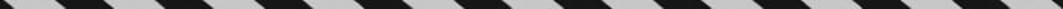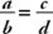﻿ ﻿FRACTIONS - Fractions, Decimals, and Percents - TOPICS IN ARITHMETIC - SAT SUBJECT TEST MATH LEVEL 1

## TOPICS IN ARITHMETIC## CHAPTER 3Fractions, Decimals, and Percents• Fractions

• Arithmetic Operations with Fractions

• Arithmetic Operations with Mixed Numbers

• Complex Fractions

• Percents

• Percent Increase and Decrease

• Exercises

Numbers such as,, andin which one integer is written over a second integer are called fractions. The center line is called the fraction bar. The integer above the fraction bar is called the numerator, and the integer below the fraction bar is called the denominator.

### FRACTIONS

Most of the numbers that we deal with in life are fractions—or can be expressed in fractions. The correct mathematical term for such a number is a rational number. A rational number is any number that can be written in the form, where a and b are integers. When a number is actually written as, we call it a fraction. For example, of 4, 0.7,, 20%, and, onlyis a fraction. However, all of them are rational numbers because they can be expressed as fractions:,,, and.

Numbers that cannot be expressed as fractions are called irrational numbers. Any nonterminating, nonrepeating decimal is an irrational number. For the Math 1 test, it is sufficient to know that π is irrational, as is, unless a = bn where b is rational. For example,is rational because 8 = 23and 2 is rational, butis irrational because there is no rational number whose square is 8.

A fraction is in lowest terms if no single positive integer greater than 1 is a factor of both the numerator and denominator. For example,is in lowest terms since no integer greater than 1 is a factor of both 8 and 15; butis not in lowest terms since 2 is a factor of both 8 and 18.

Key Fact B1

Every fraction can be reduced to lowest terms by dividing the numerator and the denominator by their greatest common factor (GCF). If the GCF is 1, the fraction is already in lowest terms.

Smart Strategy

You can always use your calculator to reduce a fraction to lowest terms.

For example, by dividing 8 and 18 by 2, their GCF, we can reduceto. Since the GCF of 4 and 9 is 1,is in lowest terms.

Key Fact B2

Every fraction can be expressed as a decimal (or a whole number) by dividing the numerator by the denominator.

If a fraction is written in lowest terms and if the only prime factors of the denominator are 2 or 5, the decimal terminates.

If a fraction is written in lowest terms and if the denominator has any prime factor other than 2 or 5, the decimal repeats.

EXAMPLE 1: Since 4, 5, 8, 10, 16, 20, 25, and 40 have no prime factors other than 2 and 5, the decimal equivalents of each of the following fractions terminate:EXAMPLE 2: Since 6, 7, 9, 12, and 22 all have prime factors other than 2 and 5 (6, 9, and 12 are multiples of 3; 7 is a multiple of 7, and 22 is a multiple of 11), the decimal equivalents of each of the following fractions repeat:To determine if two fractions are equivalent (have the same value) or if one is greater than the other, cross multiply.

Key Fact B3

To compareand, cross multiply.

If ad = bc, thenIf ad > bc, thenIf ad < bc, thenEXAMPLE 3:because. Although KEY FACT B3 is useful, remember that you can always compare fractions by using your calculator to convert them to decimals.

EXAMPLE 4:becauseand, and 0.666 > 0.625.

﻿